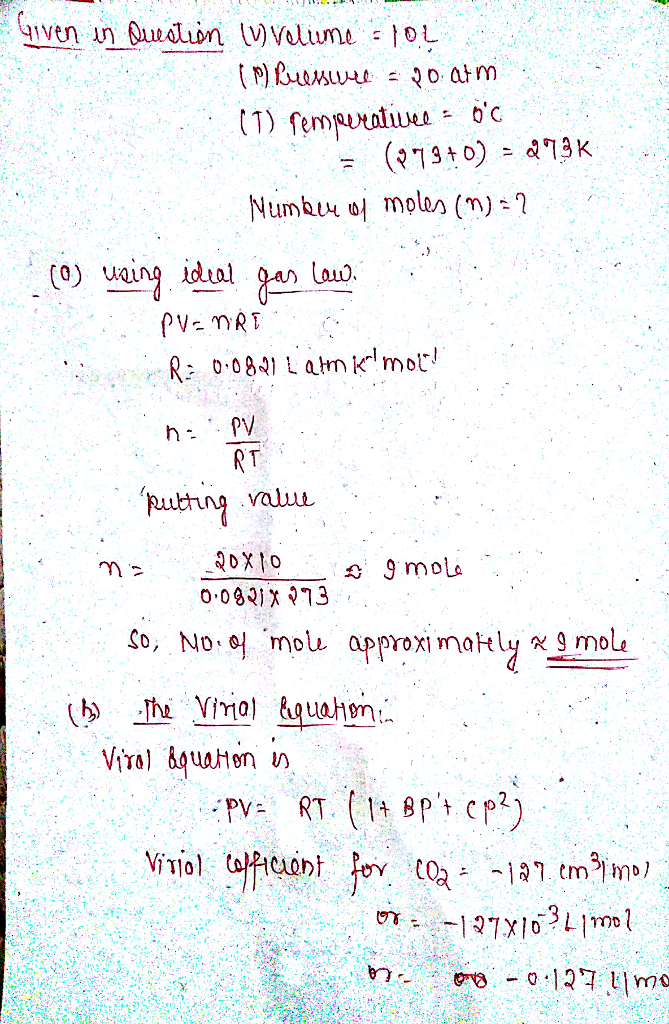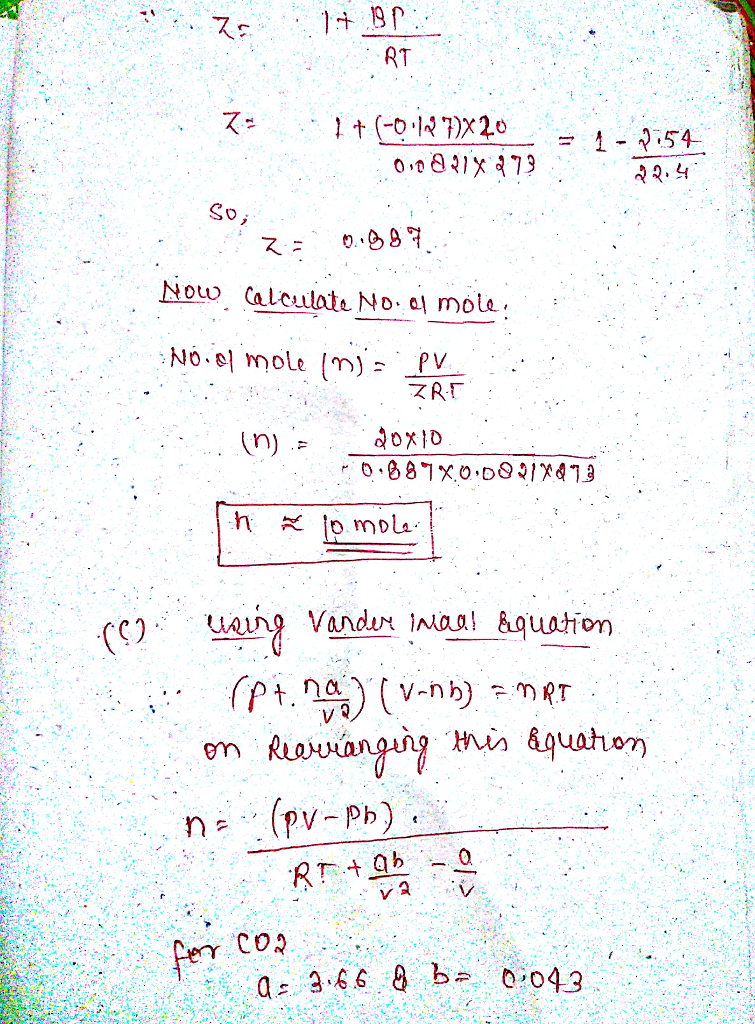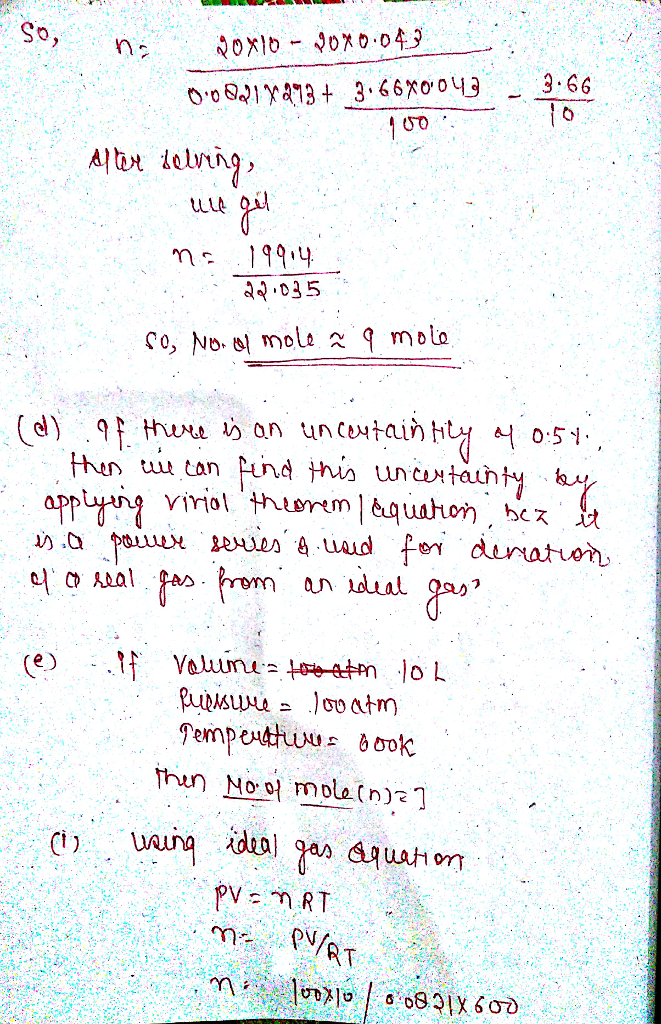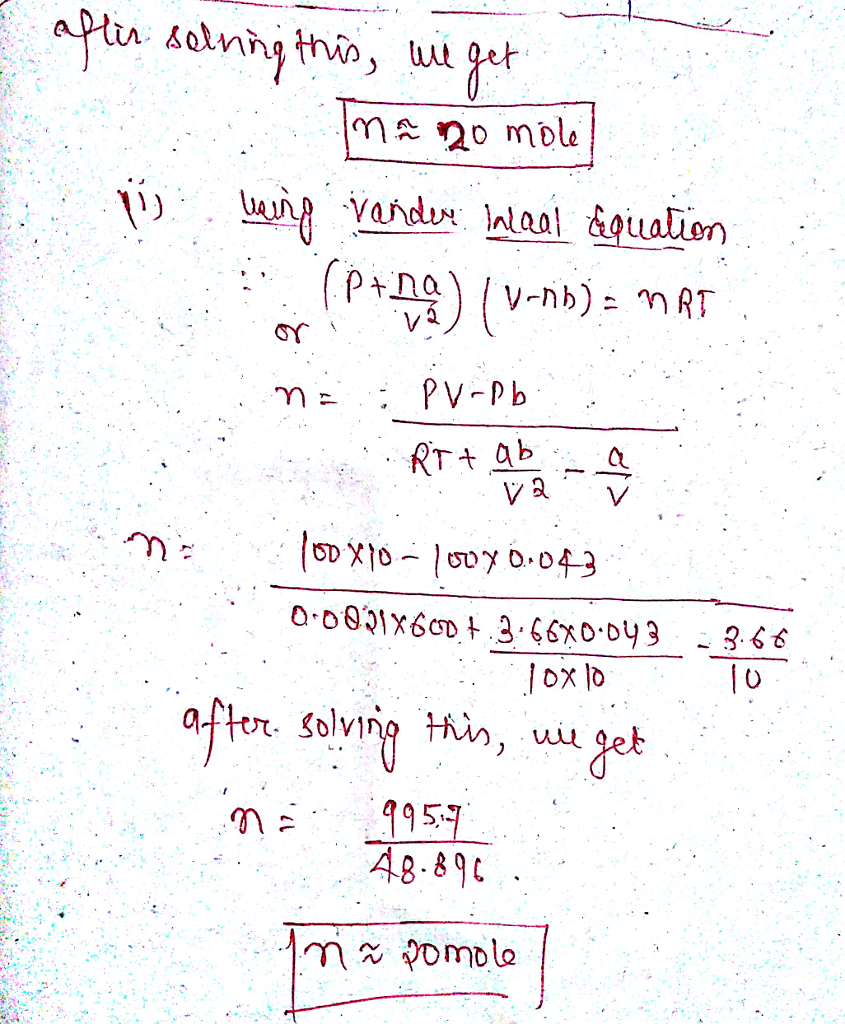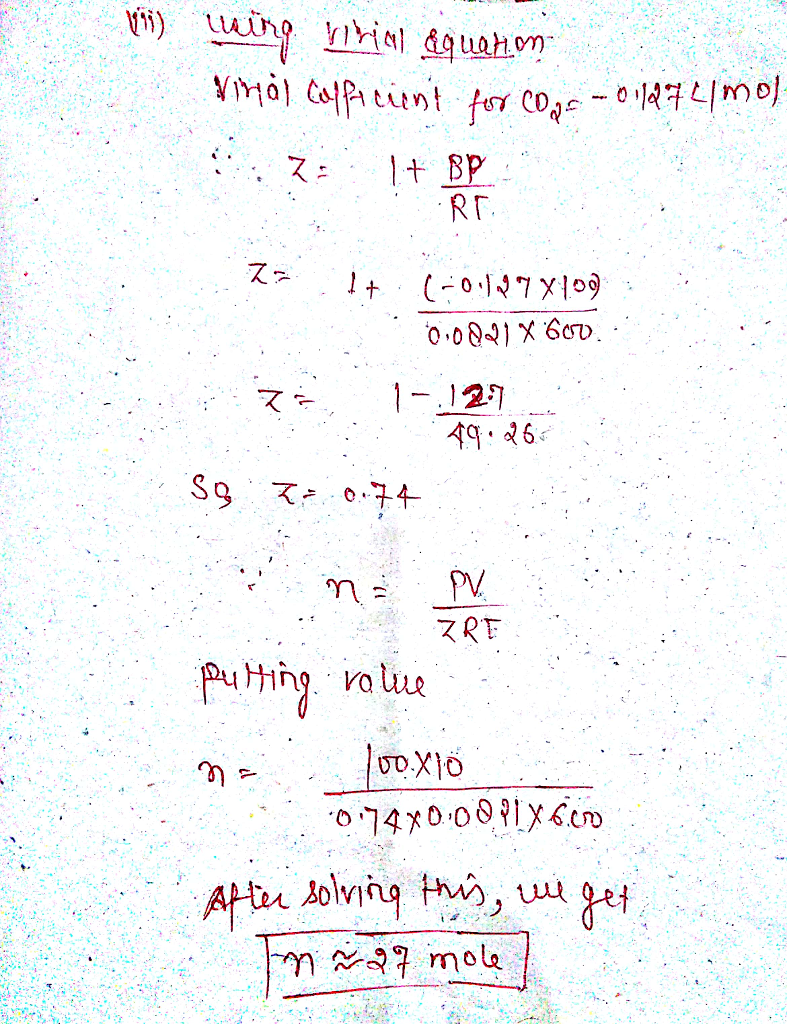# Homework Solution: 10.00L vessel containing CO2 has an internal pressure 20.0 atm, at 0°C. Calculate…

10.00L vessel containing CO2 has an internal pressure 20.0 atm, at 0°C. Calculate the number of moles of gas using: (Hint: The method might be 8th grade algebra but some computer or graphing power may be needed. The book will have the values for B (20°C), a and b for CO2). [a-c should be similar]
a. the ideal gas law
b. the virial equation
c. van der Waals equation
d. To within an uncertainty of 0.5 % does it matter which equation you use to find it? Why?
e. Would it matter if the same sample was measured 100 atm and 600K?
Can someone show be a step by step of each? Keep getting it wrong, will rate, thanks!